1) Introduction
• Phenomenon of radioactivity was first discovered by A.H.Bacquerel in 1896 while studying fluorescence and phosphorence of compounds irradiated by visible light
• these phosphorescent materials glow in dark after being exposed to visible light
• while conducting experiment on uranium salts, he found that uranium salts has a capability to blacken the photographic plate kept in a dark place wrapped through a paper
• Subsequent experiments showed that radioactivity is a nuclear phenomenon in which an unstable nucleus under goes a decay process referred as radioactive decay
• There are three types of radioactivity decays that occur in nature .These are α decay ,β decay and γ decay.
• We now define radioactive decay as the process by which unstable atomic nucleus looses energy by emitting ionizing particles or radiations ( α,β and γ rays)
• Radioactive decay of an atomic nucleus is a spontaneous process and can occur without any interaction of other particles outside the atom
• This process of radioactive decay is random and we can not predict whether a given radioactive atom will emit radiations at a particular instant of time or not
• Phenomenon of radioactivity is observed in heavy elements like uranium and unstable isotopes like carbon 14

• Radioactive rays ionize the surrounding air and affect photographic plate
• Radioactive rays acts differently on different biological cells and tissues
• A beam of radioactive rays from a radium sample into three components in presence of strong magnetic or electric fields
##### I.The alpha rays(particles)
• The alpha particles are nuclei of helium atoms
• Alpha particles was first identified by Rutherford and Royds in 1909 by spectroscopic method where they found traces of helium in an originally pure sample of Radon gas which is an α emitter.
• Examples of α decay are

(A)     222Rn864He2+218Po84

(B) 238U924He2+234Th90
• α rays can be stopped by thin sheet of paper.
• α rays can cause intense ionization in air.
• Any group of α particles emitted from same type of nuclei always have definite energy and definite velocity.
• Most α particles are emitted with velocities between ˜ 1.5x107 and ˜ 2.2x107.
• The α particles cover a definite distance in a material without any loss of intensity and suddenly in a small distance they are absorbed completely.
• The distance α rays travel within a given material is called their range in that material.
##### II.The beta rays(particles)
• β particles are identical with electrons.
• They have mass 1 ⁄ 1836 of mass of proton.
• examples of β decay are
(A)     234Th90→ 234Pa91+e-

(B) 210Bi83→ 210Po84+e-

(C) 14C6→ 14N7+e-
• Mass number and charge are conserved and the daughter product moves one place up in the periodic table, as loss of negative charge by nucleus implies gain of positive charge.
• β rays cause much less ionization in air , but are ˜ 100 times more penetrating then α rays.
• β rays can penetrate a aluminum sheet of few mm thickness.
• A particular β active element emits β particles with energies varying between zero and a certain maximum.
• This maximum energy is called end point energy.
##### III. The Gamma rays:-
• They are part of EM spectrum λγ < λX-rays
• γ ray photons are more energetic and more penetrating then X-rays photons
• λγ rages between 1.7X10-8 cm and 4.0X10-6 cm
• Ionization due to Gamma rays is a photoelectric effect
• Owing to their large energies ,the Gamma rays photons can dislodge electrons not only from outer orbits( valence orbits on conduction band) of atoms but also from the inner orbits
• Besides photoelectric effect ,gamma rays loose energy by
i) Compton effect ,in which the gamma photon collides with an electron and gets scattered with a shift in wavelengthii) Pair production ,in which a gamma photon is converted into a pair consisting of an electron and a positron( particle having mass and charge equal to electron but carrying positive change)
• Radioactivity is a nuclear phenomenon
• When a nucleus disintegrates by emitting a particle ( α and β) or by capturing an electron from the atomic shell( K-shell) ,the process is called radioactive decay. This decay process is spontaneous.
• Let us take a radioactive sample containing N0 at time t=0 i.e, at the beginning. We wish to calculate the number N of these nuclei left after time t.
• The number of nuclei of a given radioactive sample disintegrating per sec is called the activity of that sample is
dN/dt=rate of decrease of nuclei with time=Activity of sample at time t                              --(1)
• Experimentally it is found that the activity at any instant of time t is directly proportional to the number N of parent type nuclei present at that time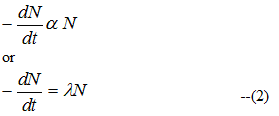Where λ > 0 is proportionality constant and negative sign indicates that N decreases as t increases
• From equation (2) we get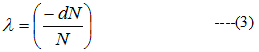i.e. ,λ is fractional change in N per sec
=> λ is not merely a proportionality constant ,but it gives us the probability of decay per unit interval of time
• Hence λ is called the probability constant or decay constant or disintegration constant
• dN is the no of parent nuclei that decay between t and t+dt and we have taken N as continuous variable
• From (2)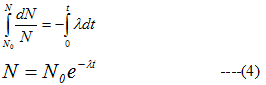N0=No of radioactive nuclei at t=0
• From (4) we see that law of radioactive decay is exponential in character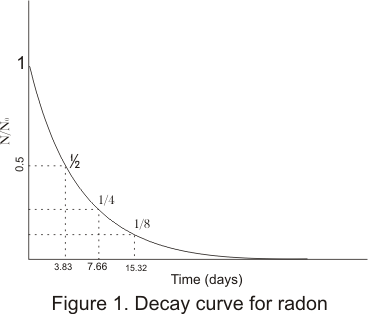• From figure it can be noted that only half the amount of radon present initially after 3.83 days and 1/4 after 7.66 days and so on
• Plot shows that in a fixed time interval a fixed fraction of the amount of radioactive substance at the beginning of interval decays
• This faction is independent of the amount of radioactive substance and depends only on the interval of the time
• The decay constant λ is a characteristics of radioactive substance and it depends in no way on the amount of the substance present
##### a) Half Life
• Time interval during which half of a given sample of radioactive substance decays is called its half life. It is denoted by T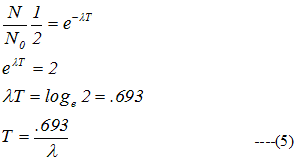##### b) Mean Life τ
• Individual radio atomic atoms may have life spans between zero and infinity
• Average or mean life τ is defined as
τ=Total life time of all nuclei in a given sample/Total no of nuclei in that sample                        --(6)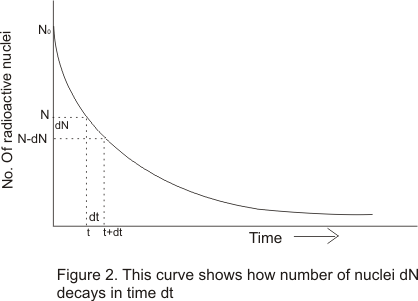• From curve one can see that each of dN number of radioactive nuclei has lived a life of t sec i.e. the total life span of a dN nuclei is (dN.t) sec . Therefore equation (6) can be written as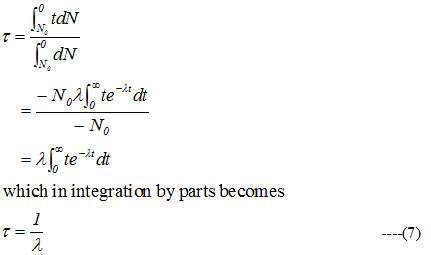4) Unit of activity
• The most commonly used unit is the curie
• Curie was originally based on the rate of decay of a gram of radium
• There are 3.7X1010 disintegrations per sec per gram of radium .This no is taken as a standard
=> One curie=3.7X1010 disintegrations per sec
• One curie of activity is very strong source of radiation
=> 1 milli curie=1mCi=10-3 Ci
1 microcurie=1μCi=10-6 Ci
• Another unit of activity is Rutherford
1rd=106 dis/sec
• Activity |dN/dt|=λN=.693N/T
=> A very short lived substance gives rise to large activity ,even it is present in minute quantities
• The SI unit of radioactivity recently proposed is Becquerel (Bq) which is defined as activity done to one disintegration per sec hence
1ci=3.7X1010 bq
=37G bq
5) Alpha decay:
• Nucleus before the decay is called parent nucleus and after the decay is called daughter nucleus
• In Alpha decay, the parent nucleus AXZ emits an α particle (=4He2) leaving behind a daughter nucleus of four mass unit less and two charge units less i.e. A-4XZ-2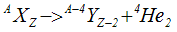• α decay shift the element two places to the left in the periodic tables of elements ex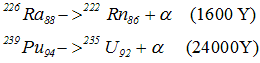• All nuclides of A >= 210 and Z > 83 tends to decay by α emission
• 209Bi is the heaviest stable nuclide in nature
• α decay in heavy nucleus occur because a too heavy nucleus becomes unstable due to coulomb repulsion and by emitting an α particle the nucleus decrease its A and Z to moves towards stability
• Now the rest mass energy of parent nucleus AXZ is greater then the sum of rest mass energies of A-4XZ-2 and 4He2
• The difference between the rest mass energies of initial constituents and final products is called Q-value of the process
• For α decay process ,Q value is
Q=[mp -(md+mα)]c2
where mp -> Mass of parent nucleus ZAX
md -> Mass of parent nucleus Z-2A-4X
mα -> Mass of parent nucleus 24He
6) β Decay
• There are two types of β decay , β- and β+
• In β decay an nucleus decay spontaneously emitting an electron or positron
• Under β- decay one of the neutrons in the parent nucleus gets transformed into a proton and in the process an electron and an antineutrino are emitted
n-> p+e--
• The daughter nucleus thus formed in β- decay would be an element one place to the right of the parent in the periodic table of elements
• Examples of β- decay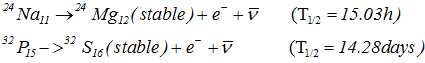• β- is common over entire range of nuclides and amongst the naturally occurring heavy radioactive nuclides and in fission products
• In β+ decay one the protons of the parent nucleus gets transformed into a neutron emitting a positron and neutrino
p->n+e+
• In β+ decay the daughter nucleus would be one place to the left of parent nuclei in the periodic table
• Examples of β+ decay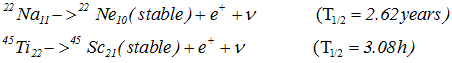• In both β+ and β- symbol ν- and ν represents antineutrino and neutrino
• Both antineutrino (ν-) and neutrino(ν) are charge less and nearly less particles and interact very weakly with matter which make their detection very difficult
• In these β decay( β+ and β-) mass number A of nucleus remain same after the decay
7) γ Decay
• After alpha or beta decay processes it is common to find the daughter nucleus to be in an excited state
• Just like atoms ,nucleus also have energy levels
• So an nucleus in excited state can make transitions from higher energy levels to lower one by the emission of electro magnetic radiation
• The energy difference in allowed energy levels of a nucleus are of the order of Mev and the photons emitted by nuclei have energies of the order of Mev and are called γ rays
• As an example,β decay of 60Co27 nucleus gets transformed into 60Ni28 nucleus in excited state which then de -excites to its ground state by successive emission of 1.17 Mev and 1.33 Mev gamma rays as shown in energy level diagram,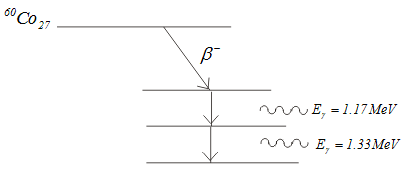No ratings yet - be the first to rate this.